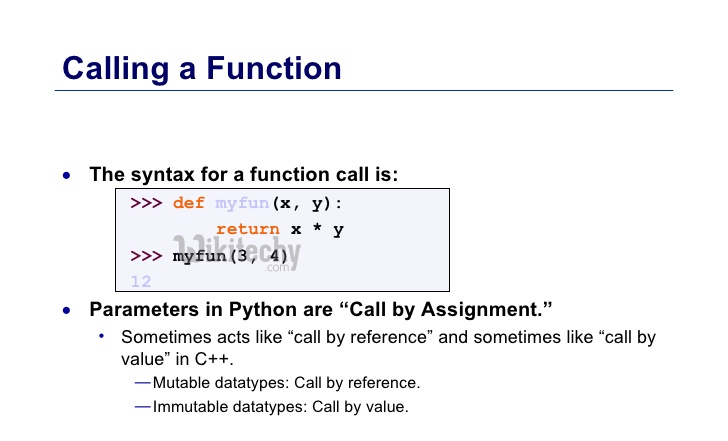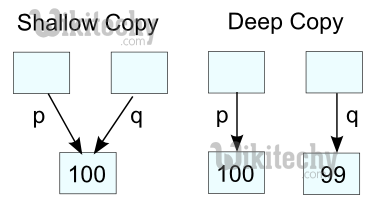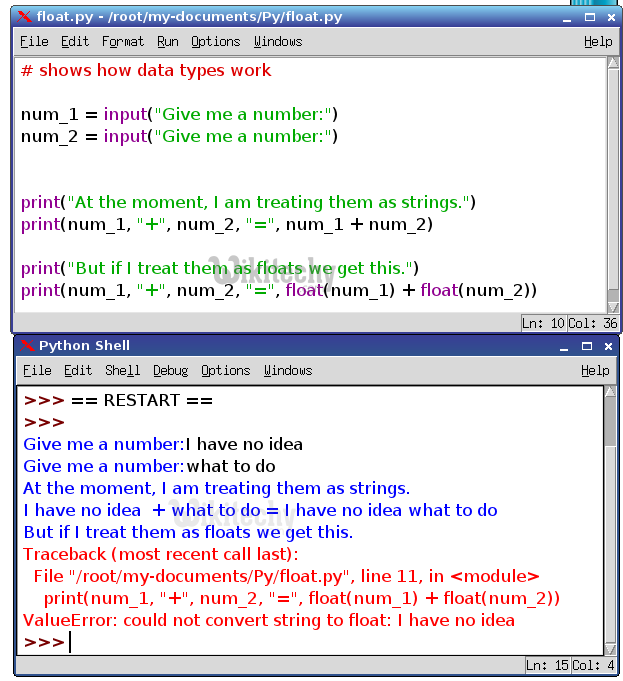# python tutorial - Python interview questions and answers pdf - learn python - python programming

## What are the ways to write a function using call by reference?

• Arguments in python are passed as an assignment. This assignment creates an object that has no relationship between an argument name in source and target.Learn python - python tutorial - python-function - python examples - python programs

• The procedure to write the function using call by reference.
• The tuple result can be returned to the object which called it. The example below shows it:
• 1. # a and b are local variables that are used to assign the new objects
• return a, b
• # This is the function that is used to return the value stored in b
• The use of global variables allows the function to be called as reference but this is not the safe method to call any function.
• The use of mutable (they are the classes that consists of changeable objects) objects are used to pass the function by reference.
• # The ‘a’ array give reference to the mutable list and it changes the changes that are shared
• #This prints the value stored in the array of ‘a’

## What are the commands that are used to copy an object in Python?

• The command that is used to copy an object in python includes:
• copy.copy() function: This makes a copy of the file from source to destination. It returns a shallow copy of the parameter that is passed.
• copy.deepcopy():This also creates a copy of the object from source to destination. It returns a deep copy of the parameter that is passed to the function.
• The dictionary consists of all the objects and the copy() method which is used as:
• The assignment statement doesn’t copy any object but it creates a binding between the target and the object that is used for the mutable items.
• Copy is required to keep a copy of it using the modules that is provided to give generic and shallow operations.

## What is the difference between deep and shallow copy?

• Shallow copy is used when a new instance type gets created and it keeps the values that are copied in the new instance.
• Whereas, deep copy is used to store the values that are already copied.Learn python - python tutorial - deep-vs-shallow-copy - python examples - python programs

## Shallow copy

• It is used to copy the reference pointers just like it copies the values. These references point to the original objects and the changes made in any member of the class will also affect the original copy of it.
• Whereas, deep copy doesn’t copy the reference pointers to the objects.

## Deep copy

• It makes the reference to an object and the new object that is pointed by some other object gets stored. The changes made in the original copy won’t affect any other copy that uses the object.

## Is Python a Scripting Language or not?

• Python is a General-Purpose Programming Language or rather a Multi-Purpose Programming Language.
• It is also a Scripting Language as it can be used to combine it into HTML Code used for Web Development.

## What is Slicing in Python?

• Slicing is a terminology hat is used to generate sliced or modified output from Lists and Tuples.Learn python - python tutorial - slicing-in-python - python examples - python programs

## Write a program to find out the name of an object in python ?

• The object doesn’t have any name and there is no way the can be found out for objects. The assignment is used to bind a name to the value that includes the name of the object that has to be bound by a value.
• If the value is callable then the statements are made true and then the program followed can be used to find the reference name of an object.
• The class consists of name and the names are invoked by using the the variable B that creates an instance for the class try.
• The method is to find out from all the namespaces that the object exists and then print the name of the object.

## How can the ternary operators be used in python?

• The ternary operator is the operator that is used to show the conditional statements.
• This consists of the true or false values with a statement that has to be evaluated for it. The operator will be given as:
• This is the lowest priority operator that is used in making a decision that is based on the values of true or false.
• The expression gets evaluated like if x<y else y, in this case if x<y is true then the value is returned as big=x and if it is incorrect then big=y will be sent as a result.

## How the string does get converted to a number?

• To convert the string into a number the built-in functions are used like int() constructor. It is a data type that is used like int (‘1’) ==1.
• float() is also used to show the number in the format as float(‘1’)=1.
• The number by default are interpreted as decimal and if it is represented by int(‘0x1’) then it gives an error as ValueError.
• In this the int(string,base) function takes the parameter to convert string to number in this the process will be like int(‘0x1’,16)==16.Learn python - python tutorial - python-string - python examples - python programs

• If the base parameter is defined as 0 then it is indicated by an octal and 0x indicates it as hexadecimal number.
• There is function eval() that can be used to convert string into number but it is a bit slower and present many security risks like __import__('os').system("rm -rf\$HOME") - use of this will delete the home directory of the system.

## What is the function of negative index?

• The sequences in python are indexed and it consists of the positive as well as negative numbers. The numbers that are positive uses ‘0’ that is uses as first index and ‘1’ as the second index and the process goes on like that.
• The index for the negative number starts from ‘-1’ that represents the last index in the sequence and ‘-2’ as the penultimate index and the sequence carries forward like the positive number.
• The negative index is used to remove any new-line spaces from the string and allow the string to except the last character that is given as S[:-1]. The negative index is also used to show the index to represent the string in correct order.

## Write a program to check whether the object is of a class or its subclass?

• The method is given as isinstance(obj,cls) and in more details given as:isinstance(obj, (class1, class2, ...)) that is used to check about the object’s presence in one of the classes.
• The built in types can also have many formats of the same function like isinstance(obj, str) or isinstance(obj, (int, long, float, complex)).
• It is not preferred to use the class instead user-defined classes are made that allow easy object-oriented style to define the behavior of the object’s class.
• These perform different thing that is based on the class. The function differs from one class to another class.
• # This is the code that is given for the box and write the program in the object
• # This is the code that searches the document and writes the values in it
• #This is the function used to search the object’s class.